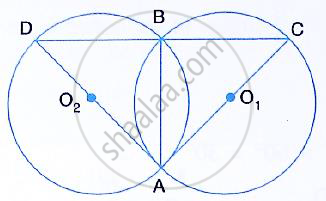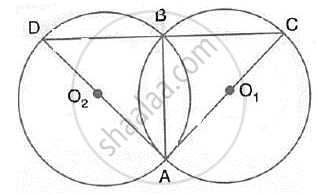Share

# In the Figure, Ab is Common Chord of the Two Circle. If Ac and Ad Are Diameters; Prove that D, B and C Are in a Straight Line. O1 and O2 Are the Centres of Two Circles. - Mathematics

Course

#### Question

In the figure, AB is common chord of the two circle. If AC and AD are diameters; prove that D,
B and C are in a straight line. O1 and O2 are the centres of two circles.#### Solution∠DBA = 90° and ∠CBA = 90°

(Angles in a semicircle is a right angle) Adding both we get,
∠DBC = 180°
∴ D, B and C form a straight line.

Is there an error in this question or solution?